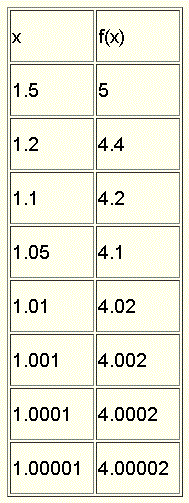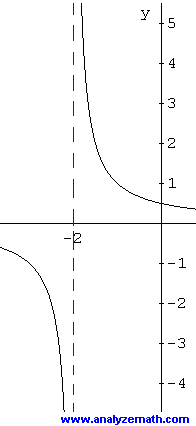# Introduction to Limits in Calculus

Numerical and graphical approaches are used to introduce to the concept of limits using examples.

## Example 1

Let f(x) = 2 x + 2 and compute f(x) as x takes values closer to 1. We first consider values of x approaching 1 from the left (x < 1).We now consider x approaching 1 from the right (x > 1).In both cases as x approaches 1, f(x) approaches 4. Intuitively, we say that limx→1 f(x) = 4.
NOTE: We are talking about the values that f(x) takes when x gets closer to 1 and not f(1). In fact we may talk about the limit of f(x) as x approaches a even when f(a) is undefined.

## Example 2

Let g(x) = sin x / x and compute g(x) as x takes values closer to 0. We consider values of x approaching 0 from the left (x < 0) and values of x approaching 0 from the right (x > 0).Here we say that limx→0 g(x) = 1. Note that g(0) is undefined.

## Example 3

The graph below shows that as x approaches 1 from the left, y = f(x) approaches 2 and this can be written as
limx→1- f(x) = 2
As x approaches 1 from the right, y = f(x) approaches 4 and this can be written as
limx→1+ f(x) = 4
Note that the left and right hand limits and f(1) = 3 are all different.## Example 4

This graph shows that
limx→1- f(x) = 2
As x approaches 1 from the right, y = f(x) approaches 4 and this can be written as
limx→1+ f(x) = 4
Note that the left hand limit and f(1) = 2 are equal.## Example 5

This graph shows that
limx→0- f(x) = 1
and
limx→0+ f(x) = 1
Note that the left and right hand limits are equal and we can write
limx→0 f(x) = 1
In this example, the limit when x approaches 0 is equal to f(0) = 1.## Example 6

This graph shows that as x approaches - 2 from the left, f(x) gets smaller and smaller without bound and there is no limit. We write
limx→-2- f(x) = - ∞
As x approaches - 2 from the right, f(x) gets larger and larger without bound and there is no limit. We write
limx→-2+ f(x) = + ∞
Note that - ∞ and + ∞ are symbols and not numbers. These are symbols used to indicate that the limit does not exist.## Example 7

The graph below shows a periodic function whose range is given by the interval [-1 , 1]. If x is allowed to increase without bound, f(x) take values within [-1 , 1] and has no limit. This can be written
limx→ + ∞ f(x) = does not exist
If x is allowed to decrease without bound, f(x) take values within [-1 , 1] and has no limit again. This can be written
limx→ - ∞ f(x) = does not exist## Example 8

If x is allowed to increase without bound, f(x) in the graph below approaches 2. This can be written
limx→ + ∞ f(x) = 2
If x is allowed to decrease without bound, f(x) approaches 2. This can be written
limx→ - ∞ f(x) = 2